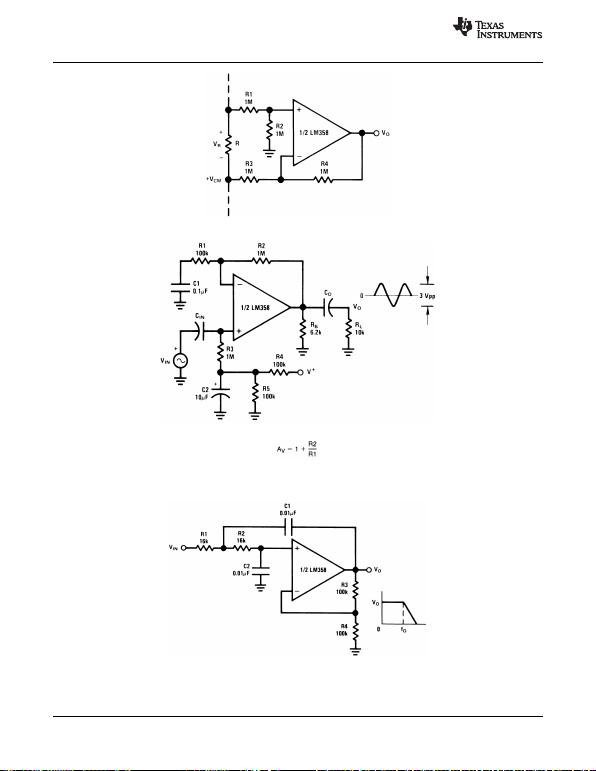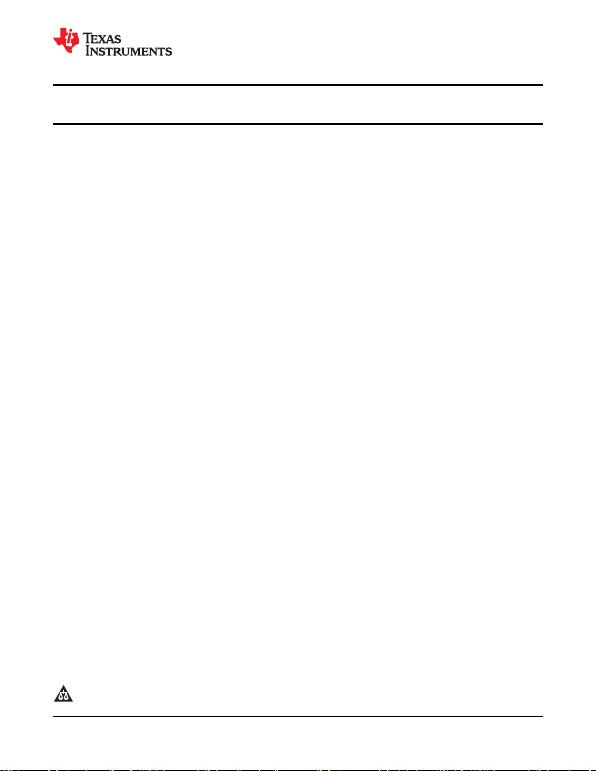LM158QML
www.ti.com
SNOSAP3F JULY 2005REVISED MARCH 2013
LM158QML Low Power Dual Operational Amplifiers
Check for Samples: LM158QML
1FEATURES Two Internally Compensated Op Amps
2 Available with Radiation Specification Eliminates Need for Dual Supplies
High Dose Rate 100 krad(Si) Allows Direct Sensing Near Gnd and VOalso
ELDRS Free 100 krad(Si) Goes to Gnd
Internally Frequency Compensated for Unity Compatible with all Forms of Logic
Gain Power Drain Suitable for Battery Operation
Large DC Voltage Gain: 100 dB
Wide Bandwidth (Unity Gain): 1 MH DESCRIPTION
z(Temperature Compensated) The LM158 series consists of two independent, high
Wide Power Supply Range: gain, internally frequency compensated operational
amplifiers which were designed specifically to operate
Single Supply: 3V to 32V from a single power supply over a wide range of
Or Dual Supplies: ±1.5V to ±16V voltages. Operation from split power supplies is also
Very Low Supply Current Drain (500 μA) possible and the low power supply current drain is
independent of the magnitude of the power supply
Essentially Independent of Supply Voltage voltage.
Low Input Offset Voltage: 2 mV Application areas include transducer amplifiers, dc
Input Common-mode Voltage Range Includes gain blocks and all the conventional op amp circuits
Ground which now can be more easily implemented in single
Differential Input Voltage Range Equal to the power supply systems. For example, the LM158
Power Supply Voltage series can be directly operated off of the standard
Large Output Voltage Swing: 0V to V+1.5V +5V power supply voltage which is used in digital
systems and will easily provide the required interface
UNIQUE CHARACTERISTICS electronics without requiring the additional ±15V
power supplies.
In the Linear Mode the Input Common-Mode
Voltage Range Includes Ground and the Output
Voltage can also Swing to Ground, even though
Operated from only a Single Power Supply
Voltage.
The Unity Gain Cross Frequency is Temperature
Compensated.
The Input Bias Current is also Temperature
Compensated.
1Please be aware that an important notice concerning availability, standard warranty, and use in critical applications of
Texas Instruments semiconductor products and disclaimers thereto appears at the end of this data sheet.
2All trademarks are the property of their respective owners.
PRODUCTION DATA information is current as of publication date. Copyright © 2005–2013, Texas Instruments Incorporated
Products conform to specifications per the terms of the Texas
Instruments standard warranty. Production processing does not
necessarily include testing of all parameters.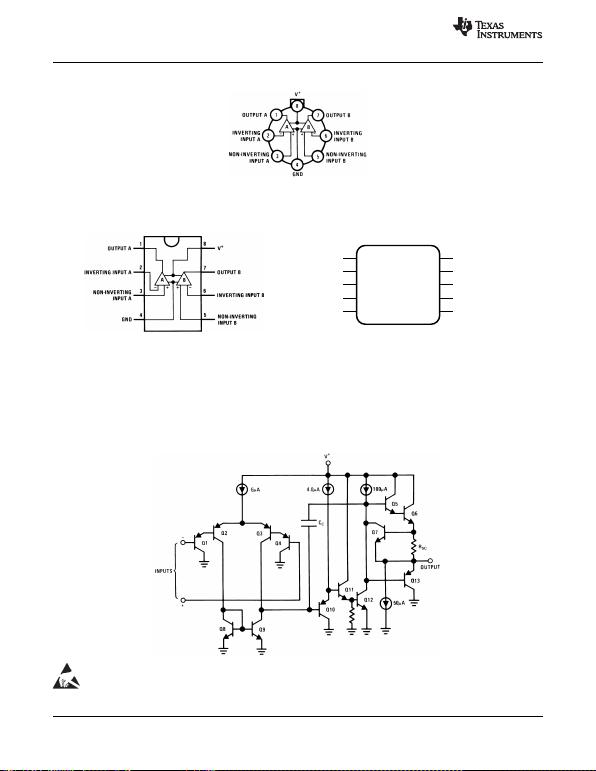OUT A V+1 10
-IN A OUT B2 9
+IN A -IN B3 8
GND +IN B4 7
N/C N/C5 6
LM158QML
SNOSAP3F JULY 2005REVISED MARCH 2013
www.ti.com
Connection Diagrams
Figure 1. TO-99 Package
See Package Number LMC0008C
Top View Top View
Figure 2. CDIP Package Figure 3. 10 Lead CLGA Package
See Package Number NAB0008A See Package Number NAC0010A
Schematic Diagram
(Each Amplifier)
These devices have limited built-in ESD protection. The leads should be shorted together or the device placed in conductive foam
during storage or handling to prevent electrostatic damage to the MOS gates.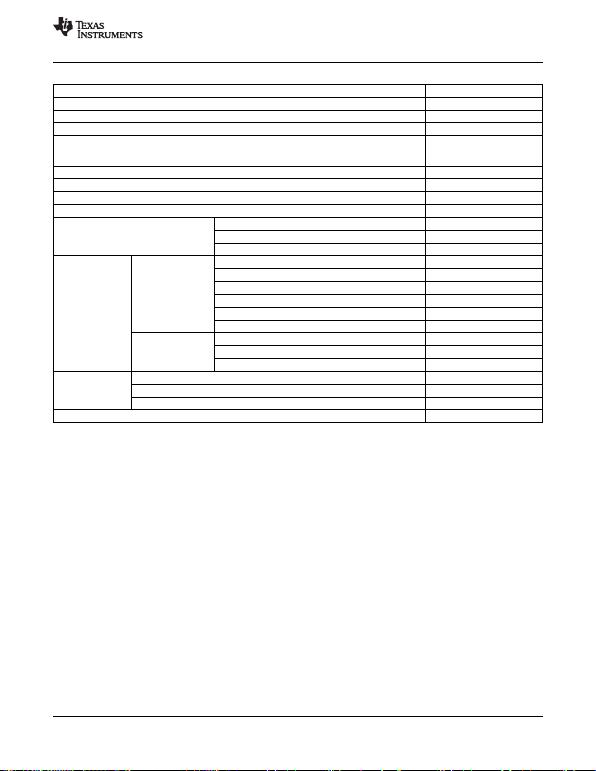LM158QML
www.ti.com
SNOSAP3F JULY 2005REVISED MARCH 2013
Absolute Maximum Ratings(1)
Supply Voltage, V+32VDC
Differential Input Voltage 32VDC
Input Voltage 0.3VDC to +32VDC
Power Dissipation(2) 830 mW
Output Short-Circuit to GND(3) Continuous
(One Amplifier)
V+15VDC and TA= 25°C
Maximum Junction Temperature (TJmax) 150°C
Input Current (VI<0.3V)(4) 50 mA
Operating Temperature Range 55°C TA+125°C
Storage Temperature Range 65°C TA+150°C
Lead Temperature (Soldering, 10 TO-99 300°C
seconds) CDIP 260°C
CLGA 260°C
Thermal θJA TO-99 (Still Air) 155°C/W
Resistance TO-99 (500LF/Min Air Flow) 80°C/W
CDIP (Still Air) 132°C/W
CDIP (500LF/Min Air Flow) 81°C/W
CLGA (Still Air) 195°C/W
CLGA (500LF/Min Air Flow) 131°C/W
θJC TO-99 42°C/W
CDIP 23°C/W
CLGA 33°C/W
Package Weight TO-99 1,000mg
CDIP 1,100mg
CLGA 220mg
ESD Tolerance(5) 250V
(1) Absolute Maximum Ratings indicate limits beyond which damage to the device may occur. Operating Ratings indicate conditions for
which the device is functional, but do not ensure specific performance limits. For ensured specifications and test conditions, see the
Electrical Characteristics. The ensured specifications apply only for the test conditions listed. Some performance characteristics may
degrade when the device is not operated under the listed test conditions.
(2) The maximum power dissipation must be derated at elevated temperatures and is dictated by TJmax (maximum junction temperature),
θJA (package junction to ambient thermal resistance), and TA(ambient temperature). The maximum allowable power dissipation at any
temperature is PDmax = (TJmax - TA)/θJA or the number given in the Absolute Maximum Ratings, whichever is lower.
(3) Short circuits from the output to V+can cause excessive heating and eventual destruction. When considering short circuits to ground,
the maximum output current is approximately 40 mA independent of the magnitude of V+. At values of supply voltage in excess of +15V,
continuous short-circuits can exceed the power dissipation ratings and cause eventual destruction. Destructive dissipation can result
from simultaneous shorts on all amplifiers.
(4) This input current will only exist when the voltage at any of the input leads is driven negative. It is due to the collector-base junction of
the input PNP transistors becoming forward biased and thereby acting as input diode clamps. In addition to this diode action, there is
also lateral NPN parasitic transistor action on the IC chip. This transistor action can cause the output voltages of the op amps to go to
the V+voltage level (or to ground for a large overdrive) for the time duration that an input is driven negative. This is not destructive and
normal output states will re-establish when the input voltage, which was negative, again returns to a value greater than 0.3V (at 25°C).
(5) Human body model, 1.5 kΩin series with 100 pF.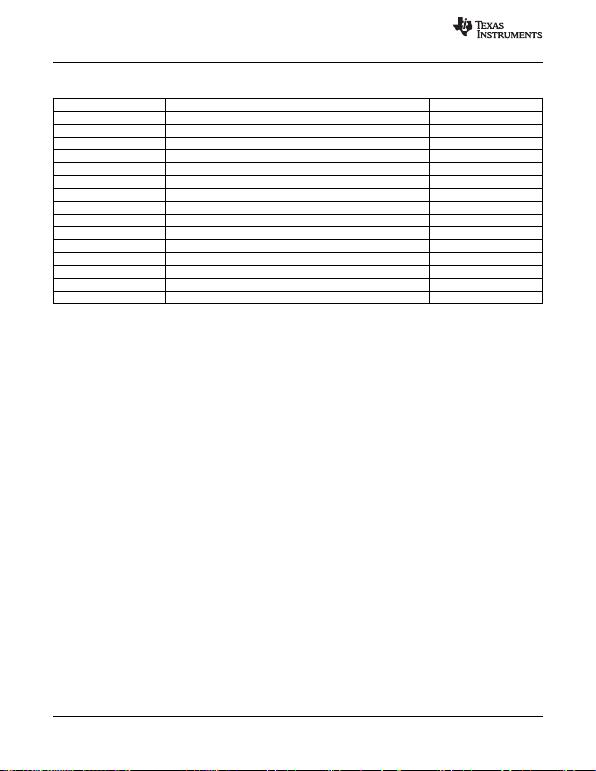LM158QML
SNOSAP3F JULY 2005REVISED MARCH 2013
www.ti.com
Quality Conformance Inspection
Mil-Std-883, Method 5005 - Group A
Subgroup Description Temp °C
1 Static tests at +25
2 Static tests at +125
3 Static tests at -55
4 Dynamic tests at +25
5 Dynamic tests at +125
6 Dynamic tests at -55
7 Functional tests at +25
8A Functional tests at +125
8B Functional tests at -55
9 Switching tests at +25
10 Switching tests at +125
11 Switching tests at -55
12 Settling time at +25
13 Settling time at +125
14 Settling time at -55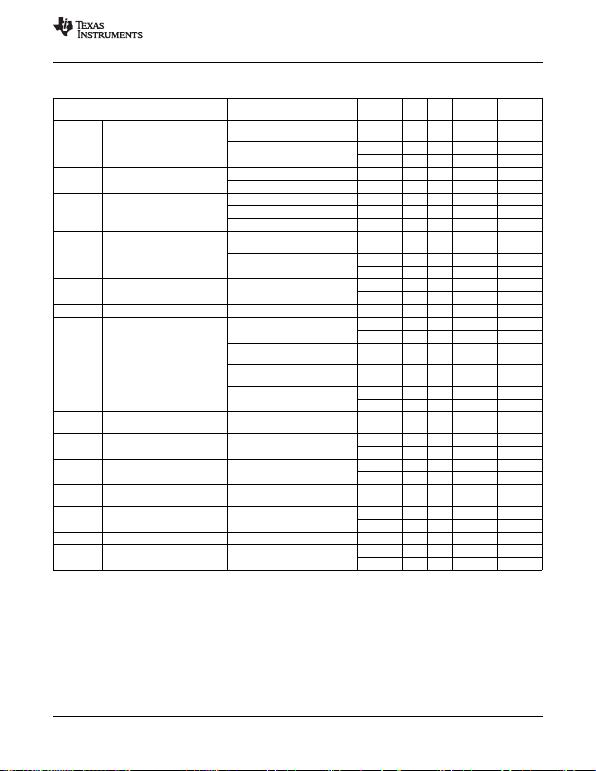LM158QML
www.ti.com
SNOSAP3F JULY 2005REVISED MARCH 2013
LM158 Electrical Characteristics SMD 5962–8771001 DC Parameters
The following conditions apply, unless otherwise specified. All voltages referenced to device ground. Sub-
Parameter Test Conditions Notes Min Max Units groups
ICC Power Supply Current +VCC = 5V, RL= 100K, 1.2 mA 1, 2, 3
VO= 1.4V
+VCC = 30V, RL= 100K, 3.0 mA 1
VO= 1.4V 4.0 mA 2, 3
VOH Output Voltage High +VCC = 30V, RL= 2K26 V 1, 2, 3
+VCC = 30V, RL= 10K27 V 1, 2, 3
VOL Output Voltage Low +VCC = 30V, RL= 10K20 mV 1, 2, 3
+VCC = 30V, ISink = 1µA 20 mV 1, 2, 3
+VCC = 5V, RL= 10K20 mV 1, 2, 3
ISink Output Sink Current +VCC = 15V, VO= 200mV, 12 µA 1
+VI= 0V, -VI= +65mV
+VCC = 15V, VO= 2V, 10 mA 1
+VI= 0V, -VI= +65mV 5.0 mA 2, 3
ISource Output Source Current +VCC = 15V, VO= 2V, -20 mA 1
+VI= 0V, -VI= -65mV -10 mA 2, 3
IOS Short Circuit Current +VCC = 5V, VO= 0V -60 mA 1
VIO Input Offset Voltage +VCC = 30V, VCM = 0V, -5.0 5.0 mV 1
RS= 50, VO= 1.4V -7.0 7.0 mV 2, 3
+VCC = 30V, VCM = 28.5V, -5.0 5.0 mV 1
RS= 50, VO= 1.4V
+VCC = 30V, VCM = 28V, -7.0 7.0 mV 2, 3
RS= 50, VO= 1.4V
+VCC = 5V, VCM = 0V, -5.0 5.0 mV 1
RS= 50, VO= 1.4V -7.0 7.0 mV 2, 3
CMRR Common Mode Rejection Ratio +VCC = 30V, RS= 5070 dB 1
VI= 0V to 28.5V,
±IIB Input BIas Current +VCC = 5V, VCM = 0V See(1) -150 -1.0 nA 1
See(1) -300 -1.0 nA 2, 3
IIO Input Offset Current +VCC = 5V, VCM = 0V -30 30 nA 1
-100 100 nA 2, 3
PSRR Power Supply Rejection Ratio +VCC = 5V to 30V, 65 dB 1
VCM = 0V
VCM Common Mode Voltage Range +VCC = 30V See(2),(3) 28.5 V 1
See(2),(3) 28.0 V 2, 3
VDiff Differential Input Voltage See(4) 32 V 1, 2, 3
AVS Large Signal Gain +VCC = 15V, RL= 2K, 50 V/mV 4
VO= 1V to 11V 25 V/mV 5, 6
(1) The direction of the input current is out of the IC due to the PNP input stage. This current is essentially constant, independent of the
state of the output so no loading change exists on the input lines.
(2) The input common-mode voltage of either input signal voltage should not be allowed to go negative by more than 0.3V (at 25°C). The
upper end of the common-mode voltage range is V+1.5V (at 25°C), but either or both inputs can go to +32V without damage,
independent of the magnitude of V+.
(3) Specified by input offset voltage.
(4) Specified parameter not tested.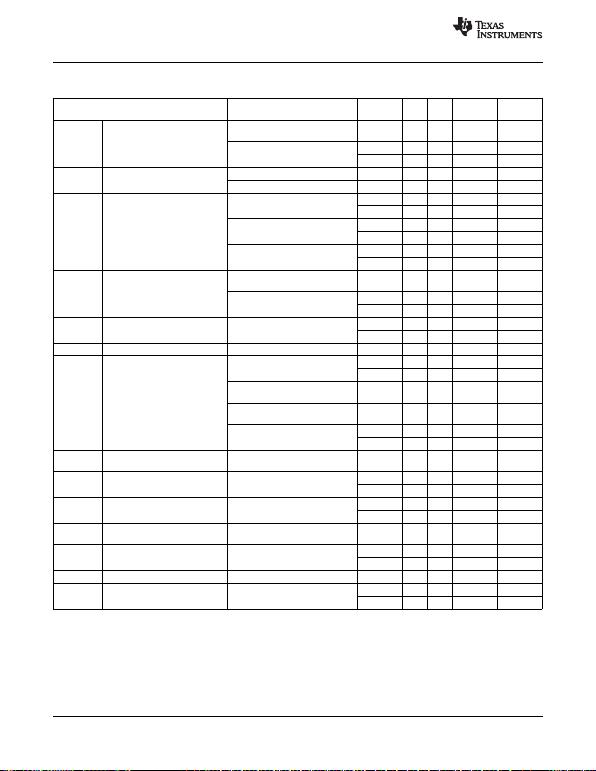LM158QML
SNOSAP3F JULY 2005REVISED MARCH 2013
www.ti.com
LM158A Electrical Characteristics SMD 5962–8771002, High Dose Rate DC Parameters
The following conditions apply, unless otherwise specified. All voltages referenced to device ground. Sub-
Parameter Test Conditions Notes Min Max Units groups
ICC Power Supply Current +VCC = 5V, RL= 100K, 1.2 mA 1, 2, 3
VO= 1.4V
+VCC = 30V, RL= 100K, 3.0 mA 1
VO= 1.4V 4.0 mA 2, 3
VOH Output Voltage High +VCC = 30V, RL= 2K26 V 1, 2, 3
+VCC = 30V, RL= 10K27 V 1, 2, 3
VOL Output Voltage Low +VCC = 30V, RL= 10K40 mV 1
100 mV 2, 3
+VCC = 30V, ISink = 1µA 40 mV 1
100 mV 2, 3
+VCC = 5V, RL= 10K40 mV 1
100 mV 2, 3
ISink Output Sink Current +VCC = 15V, VO= 200mV, 12 µA 1
+VI= 0V, -VI= +65mV
+VCC = 15V, VO= 2V, 10 mA 1
+VI= 0V, -VI= +65mV 5.0 mA 2, 3
ISource Output Source Current +VCC = 15V, VO= 2V, -20 mA 1
+VI= 0V, -VI= -65mV -10 mA 2, 3
IOS Short Circuit Current +VCC = 5V, VO= 0V -60 mA 1
VIO Input Offset Voltage +VCC = 30V, VCM = 0V, -2.0 2.0 mV 1
RS= 50, VO= 1.4V -4.0 4.0 mV 2, 3
+VCC = 30V, VCM = 28.5V, -2.0 2.0 mV 1
RS= 50, VO= 1.4V
+VCC = 30V, VCM = 28V, -4.0 4.0 mV 2, 3
RS= 50, VO= 1.4V
+VCC = 5V, VCM = 0V, -2.0 2.0 mV 1
RS= 50, VO= 1.4V -4.0 4.0 mV 2, 3
CMRR Common Mode Rejection Ratio +VCC = 30V, RS= 5070 dB 1
VI= 0V to 28.5V,
±IIB Input BIas Current +VCC = 5V, VCM = 0V See(1) -50 -1.0 nA 1
See(1) -100 -1.0 nA 2, 3
IIO Input Offset Current +VCC = 5V, VCM = 0V -10 10 nA 1
-30 30 nA 2, 3
PSRR Power Supply Rejection Ratio +VCC = 5V to 30V, 65 dB 1
VCM = 0V
VCM Common Mode Voltage Range +VCC = 30V See(2) (3) 28.5 V 1
See(2) (3) 28.0 V 2, 3
VDiff Differential Input Voltage See (4) 32 V 1, 2, 3
AVS Large Signal Gain +VCC = 15V, RL= 2K, 50 V/mV 4
VO= 1V to 11V 25 V/mV 5, 6
(1) The direction of the input current is out of the IC due to the PNP input stage. This current is essentially constant, independent of the
state of the output so no loading change exists on the input lines.
(2) The input common-mode voltage of either input signal voltage should not be allowed to go negative by more than 0.3V (at 25°C). The
upper end of the common-mode voltage range is V+1.5V (at 25°C), but either or both inputs can go to +32V without damage,
independent of the magnitude of V+.
(3) Specified by input offset voltage.
(4) Specified parameter not tested.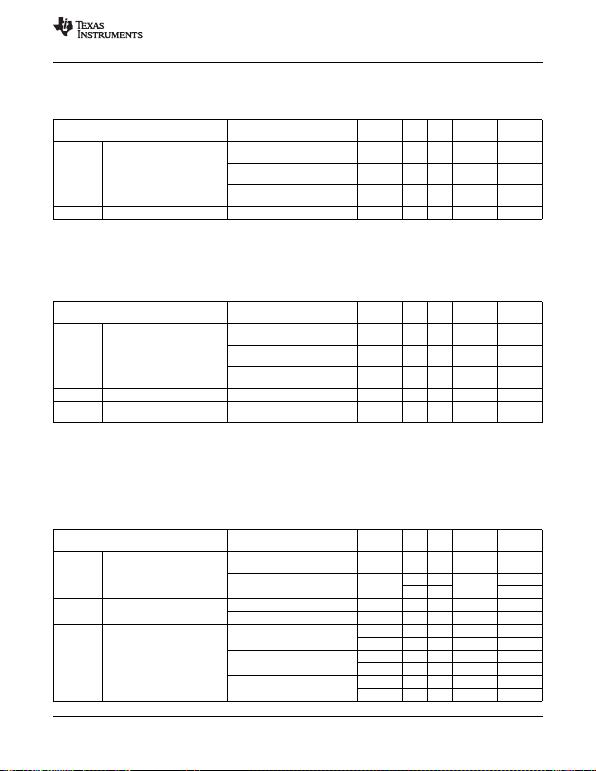LM158QML
www.ti.com
SNOSAP3F JULY 2005REVISED MARCH 2013
SMD 5962–8771002, High Dose Rate
DC Drift Parameters
The following conditions apply, unless otherwise specified. All voltages referenced to device ground.
Delta calculations are performed on QMLV devices at Group B, Subgroup 5 only. Sub-
Parameter Test Conditions Notes Min Max Units groups
VIO Input Offset Voltage +VCC = 30V, VCM = 0V, -0.5 0.5 mV 1
RS= 50, VO= 1.4V
+VCC = 30V, VCM = 28.5V, -0.5 0.5 mV 1
RS= 50, VO= 1.4V
+VCC = 5V, VCM = 0V, -0.5 0.5 mV 1
RS= 50, VO= 1.4V
±IIB Input Bias Current +VCC = 5V, VCM = 0V See(1) -10 10 nA 1
(1) The direction of the input current is out of the IC due to the PNP input stage. This current is essentially constant, independent of the
state of the output so no loading change exists on the input lines.
SMD 5962–8771002, High Dose Rate SMD 5962–8771002, High Dose Rate
100K Post Radiation Limits @ +25°C(1)
DC Parameters
The following conditions apply, unless otherwise specified. All voltages referenced to device ground.
Parameter Test Conditions Notes Min Max Units Sub -
groups
VIO Input Offset Voltage +VCC = 30V, VCM = 0V, See(1) -4.0 4.0 mV 1
RS= 50, VO= 1.4V
+VCC = 30V, VCM = 28.5V, See(1) -4.0 4.0 mV 1
RS= 50, VO= 1.4V
+VCC = 5V, VCM = 0V, See(1) -4.0 4.0 mV 1
RS= 50, VO= 1.4V
±IIB Input Bias Current +VCC = 5V, VCM = 0V See(1)(2) -60 -1.0 nA 1
ICC Power Supply Current +VCC = 5V, RL= 100K, See(1) 1.5 mA 1
VO= 1.4V
(1) Pre and post irradiation limits are identical to those listed under AC and DC electrical characteristics except as listed in the Post
Radiation Limits Table. These parts may be dose rate sensitive in a space environment and demonstrate enhanced low dose rate
sensitivity. Radiation end point limits for the noted parameters are specified only for the conditions as specified in MIL-STD-883, per
Test Method 1019, Condition A.
(2) The direction of the input current is out of the IC due to the PNP input stage. This current is essentially constant, independent of the
state of the output so no loading change exists on the input lines.
LM158A Electrical Characteristics SMD 5962–8771003 ELDRS Free Only
DC Parameters
The following conditions apply, unless otherwise specified. All voltages referenced to device ground. Sub-
Parameter Test Conditions Notes Min Max Units groups
ICC Power Supply Current +VCC = 5V, RL= 100K, 1.2 mA 1, 2, 3
VO= 1.4V
+VCC = 30V, RL= 100K, 3.0 mA 1,
VO= 1.4V 4.0 2, 3
VOH Output Voltage High +VCC = 30V, RL= 2K26 V 1, 2, 3
+VCC = 30V, RL= 10K27 V 1, 2, 3
VOL Output Voltage Low +VCC = 30V, RL= 10K40 mV 1
100 mV 2, 3
+VCC = 30V, ISink = 1µA 40 mV 1
100 mV 2, 3
+VCC = 5V, RL= 10K40 mV 1
100 mV 2, 3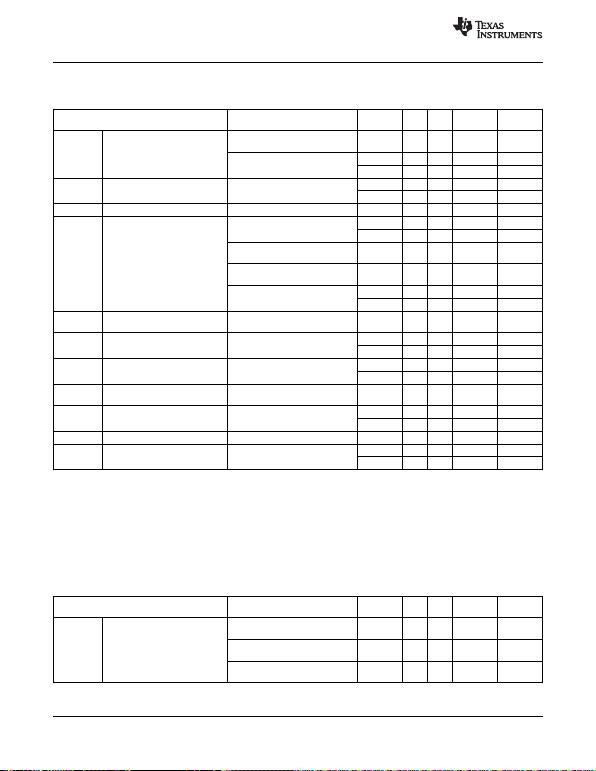LM158QML
SNOSAP3F JULY 2005REVISED MARCH 2013
www.ti.com
LM158A Electrical Characteristics SMD 5962–8771003 ELDRS Free Only
DC Parameters (continued)
The following conditions apply, unless otherwise specified. All voltages referenced to device ground. Sub-
Parameter Test Conditions Notes Min Max Units groups
ISink Output Sink Current +VCC = 15V, VO= 200mV, 12 µA 1
+VI= 0V, -VI= +65mV
+VCC = 15V, VO= 2V, 10 mA 1
+VI= 0V, -VI= +65mV 5.0 mA 2, 3
ISource Output Source Current +VCC = 15V, VO= 2V, -20 mA 1
+VI= 0V, -VI= -65mV -10 mA 2, 3
IOS Short Circuit Current +VCC = 5V, VO= 0V -60 mA 1
VIO Input Offset Voltage +VCC = 30V, VCM = 0V, -2.0 2.0 mV 1
RS= 50, VO= 1.4V -4.0 4.0 mV 2, 3
+VCC = 30V, VCM = 28.5V, -2.0 2.0 mV 1
RS= 50, VO= 1.4V
+VCC = 30V, VCM = 28V, -4.0 4.0 mV 2, 3
RS= 50, VO= 1.4V
+VCC = 5V, VCM = 0V, -2.0 2.0 mV 1
RS= 50, VO= 1.4V -4.0 4.0 mV 2, 3
CMRR Common Mode Rejection Ratio +VCC = 30V, RS= 5070 dB 1
VI= 0V to 28.5V,
±IIB Input BIas Current +VCC = 5V, VCM = 0V See(1) -50 -1.0 nA 1
See(1) -100 -1.0 nA 2, 3
IIO Input Offset Current +VCC = 5V, VCM = 0V -10 10 nA 1
-30 30 nA 2, 3
PSRR Power Supply Rejection Ratio +VCC = 5V to 30V, 65 dB 1
VCM = 0V
VCM Common Mode Voltage Range +VCC = 30V See(2),(3) 28.5 V 1
See(2),(3) 28.0 V 2, 3
VDiff Differential Input Voltage See(4) 32 V 1, 2, 3
AVS Large Signal Gain +VCC = 15V, RL= 2K, 50 V/mV 4
VO= 1V to 11V 25 V/mV 5, 6
(1) The direction of the input current is out of the IC due to the PNP input stage. This current is essentially constant, independent of the
state of the output so no loading change exists on the input lines.
(2) The input common-mode voltage of either input signal voltage should not be allowed to go negative by more than 0.3V (at 25°C). The
upper end of the common-mode voltage range is V+1.5V (at 25°C), but either or both inputs can go to +32V without damage,
independent of the magnitude of V+.
(3) Specified by input offset voltage.
(4) Specified parameter not tested.
SMD 5962–8771003 ELDRS Free Only
DC Drift Parameters
The following conditions apply, unless otherwise specified. All voltages referenced to device ground.
Delta calculations are performed on QMLV devices at Group B, Subgroup 5 only. Sub-
Parameter Test Conditions Notes Min Max Units groups
VIO Input Offset Voltage +VCC = 30V, VCM = 0V, -0.5 0.5 mV 1
RS= 50, VO= 1.4V
+VCC = 30V, VCM = 28.5V, -0.5 0.5 mV 1
RS= 50, VO= 1.4V
+VCC = 5V, VCM = 0V, -0.5 0.5 mV 1
RS= 50, VO= 1.4V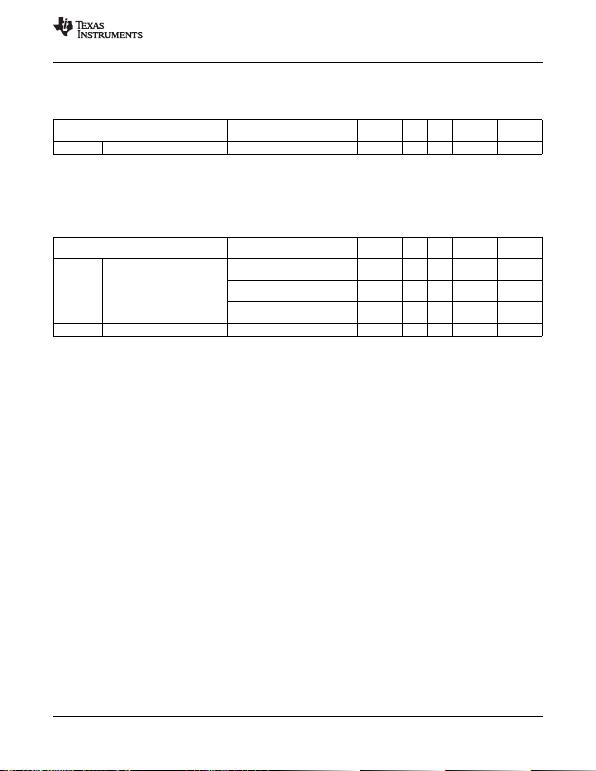LM158QML
www.ti.com
SNOSAP3F JULY 2005REVISED MARCH 2013
SMD 5962–8771003 ELDRS Free Only
DC Drift Parameters (continued)
The following conditions apply, unless otherwise specified. All voltages referenced to device ground.
Delta calculations are performed on QMLV devices at Group B, Subgroup 5 only. Sub-
Parameter Test Conditions Notes Min Max Units groups
±IIB Input Bias Current +VCC = 5V, VCM = 0V See(1) -10 10 nA 1
(1) The direction of the input current is out of the IC due to the PNP input stage. This current is essentially constant, independent of the
state of the output so no loading change exists on the input lines.
SMD 5962–8771003 ELDRS Free Only
100K Post Radiation Limits @ +25°C(1)
DC Parameters
The following conditions apply, unless otherwise specified. All voltages referenced to device ground.
Parameter Test Conditions Notes Min Max Units Sub -
groups
VIO Input Offset Voltage +VCC = 30V, VCM = 0V, See(1) -4.0 4.0 mV 1
RS= 50, VO= 1.4V
+VCC = 30V, VCM = 28.5V, See(1) -4.0 4.0 mV 1
RS= 50, VO= 1.4V
+VCC = 5V, VCM = 0V, See(1) -4.0 4.0 mV 1
RS= 50, VO= 1.4V
±IIB Input Bias Current +VCC = 5V, VCM = 0V See(1)(2) -60 -1.0 nA 1
(1) Pre and post irradiation limits are identical to those listed under AC and DC electrical characteristics except as listed in the Post
Radiation Limits Table. These parts may be sensitive in a high dose environment. Low dose rate testing has been performed on a
wafer-by-wafer basis, per Test Method 1019, Condition D of MIL-STD-883, with no enhanced low dose rate sensitivity (ELDRS).
(2) The direction of the input current is out of the IC due to the PNP input stage. This current is essentially constant, independent of the
state of the output so no loading change exists on the input lines.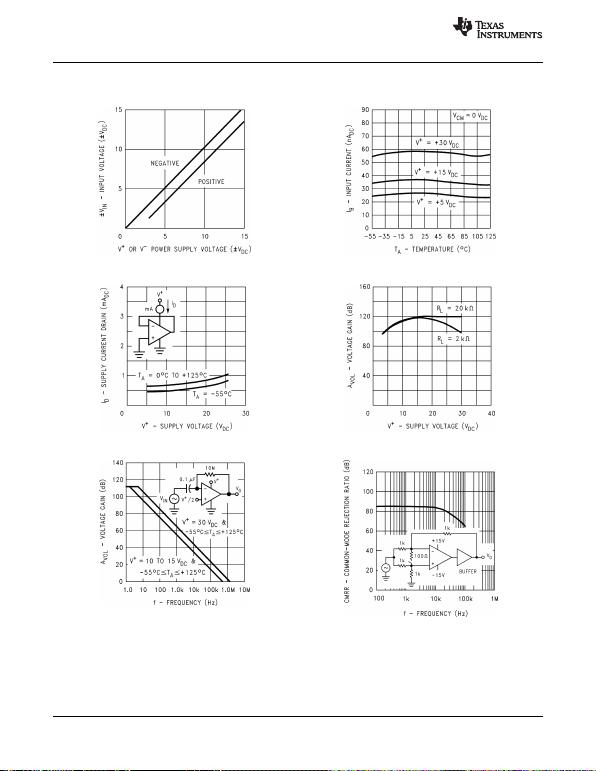LM158QML
SNOSAP3F JULY 2005REVISED MARCH 2013
www.ti.com
Typical Performance Characteristics
Input Voltage Range Input Current
Figure 4. Figure 5.
Supply Current Voltage Gain
Figure 6. Figure 7.
Open Loop Frequency Response Common-Mode Rejection Ratio
Figure 8. Figure 9.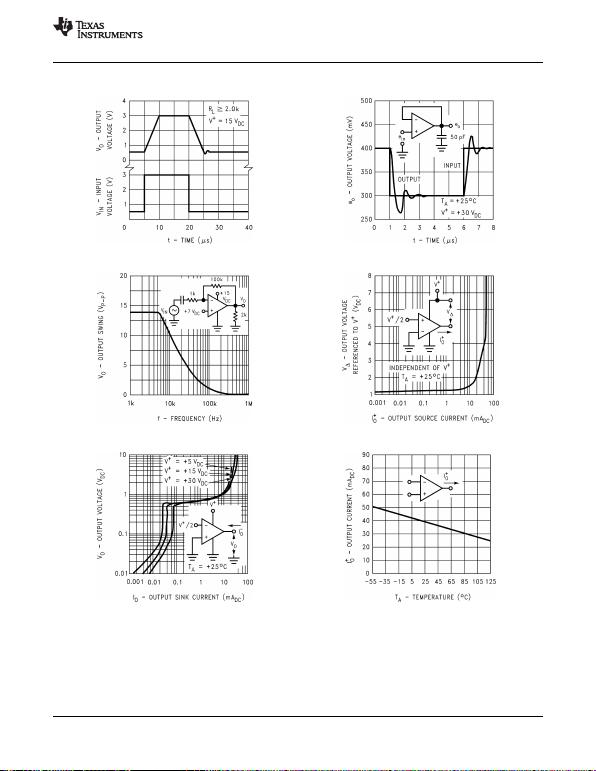LM158QML
www.ti.com
SNOSAP3F JULY 2005REVISED MARCH 2013
Typical Performance Characteristics (continued)
Voltage Follower Pulse Response Voltage Follower Pulse Response (Small Signal)
Figure 10. Figure 11.
Large Signal Frequency Response Output Characteristics Current Sourcing
Figure 12. Figure 13.
Output Characteristics Current Sinking Current Limiting
Figure 14. Figure 15.LM158QML
SNOSAP3F JULY 2005REVISED MARCH 2013
www.ti.com
APPLICATION HINTS
The LM158 series are op amps which operate with only a single power supply voltage, have true-differential
inputs, and remain in the linear mode with an input common-mode voltage of 0 VDC. These amplifiers operate
over a wide range of power supply voltage with little change in performance characteristics. At 25°C amplifier
operation is possible down to a minimum supply voltage of 2.3 VDC.
Precautions should be taken to insure that the power supply for the integrated circuit never becomes reversed in
polarity or that the unit is not inadvertently installed backwards in a test socket as an unlimited current surge
through the resulting forward diode within the IC could cause fusing of the internal conductors and result in a
destroyed unit.
Large differential input voltages can be easily accommodated and, as input differential voltage protection diodes
are not needed, no large input currents result from large differential input voltages. The differential input voltage
may be larger than V+without damaging the device. Protection should be provided to prevent the input voltages
from going negative more than 0.3 VDC (at 25°C). An input clamp diode with a resistor to the IC input terminal
can be used.
To reduce the power supply current drain, the amplifiers have a class A output stage for small signal levels which
converts to class B in a large signal mode. This allows the amplifiers to both source and sink large output
currents. Therefore both NPN and PNP external current boost transistors can be used to extend the power
capability of the basic amplifiers. The output voltage needs to raise approximately 1 diode drop above ground to
bias the on-chip vertical PNP transistor for output current sinking applications.
For ac applications, where the load is capacitively coupled to the output of the amplifier, a resistor should be
used, from the output of the amplifier to ground to increase the class A bias current and prevent crossover
distortion. Where the load is directly coupled, as in dc applications, there is no crossover distortion.
Capacitive loads which are applied directly to the output of the amplifier reduce the loop stability margin. Values
of 50 pF can be accommodated using the worst-case non-inverting unity gain connection. Large closed loop
gains or resistive isolation should be used if larger load capacitance must be driven by the amplifier.
The bias network of the LM158 establishes a drain current which is independent of the magnitude of the power
supply voltage over the range of 3 VDC to 30 VDC.
Output short circuits either to ground or to the positive power supply should be of short time duration. Units can
be destroyed, not as a result of the short circuit current causing metal fusing, but rather due to the large increase
in IC chip dissipation which will cause eventual failure due to excessive junction temperatures. Putting direct
short-circuits on more than one amplifier at a time will increase the total IC power dissipation to destructive
levels, if not properly protected with external dissipation limiting resistors in series with the output leads of the
amplifiers. The larger value of output source current which is available at 25°C provides a larger output current
capability at elevated temperatures (see Typical Performance Characteristics) than a standard IC op amp.
The circuits presented in the section on typical applications emphasize operation on only a single power supply
voltage. If complementary power supplies are available, all of the standard op amp circuits can be used. In
general, introducing a pseudo-ground (a bias voltage reference of V+/2) will allow operation above and below this
value in single power supply systems. Many application circuits are shown which take advantage of the wide
input common-mode voltage range which includes ground. In most cases, input biasing is not required and input
voltages which range to ground can easily be accommodated.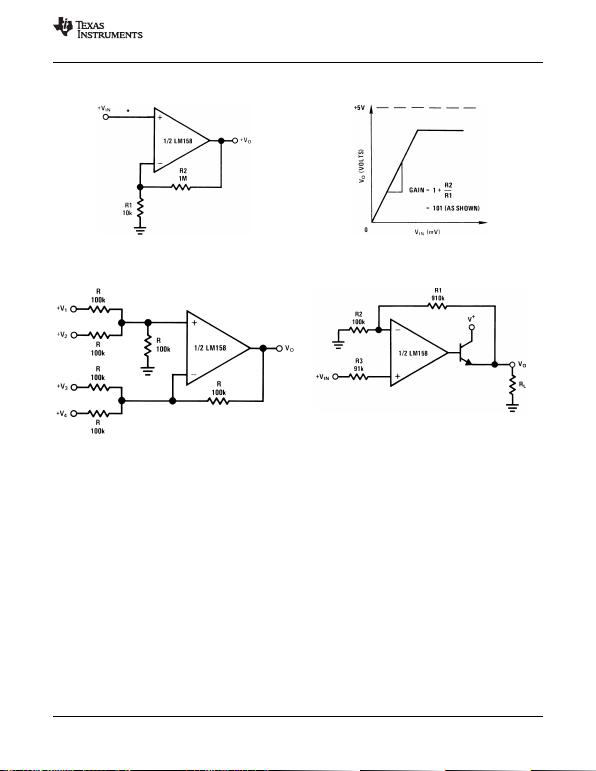LM158QML
www.ti.com
SNOSAP3F JULY 2005REVISED MARCH 2013
Typical Single-Supply Applications
(V+= 5.0 VDC)
*R not needed due to temperature independent IIN
Figure 16. Non-Inverting DC Gain (0V Output)
VO= 0 VDC for VIN = 0 VDC
AV= 10
Where: VO= V1+ V2V3V4
(V1+ V2)(V3+ V4) to keep VO> 0 VDC
Figure 17. DC Summing Amplifier Figure 18. Power Amplifier
(VIN'S 0 VDC and VO0 VDC)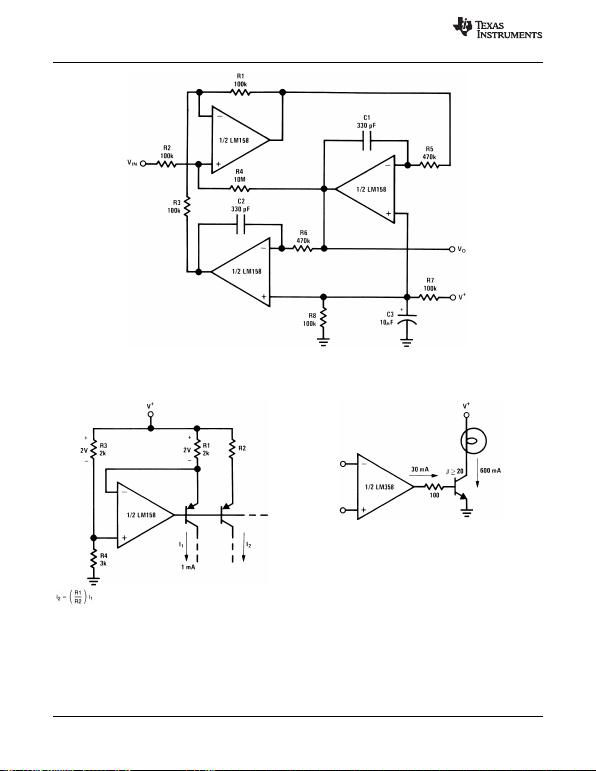LM158QML
SNOSAP3F JULY 2005REVISED MARCH 2013
www.ti.com
fo= 1 kHz
Q = 50
Av= 100 (40 dB) Figure 19. “BI-QUAD” RC Active Bandpass Filter
Figure 20. Fixed Current Sources Figure 21. Lamp Driver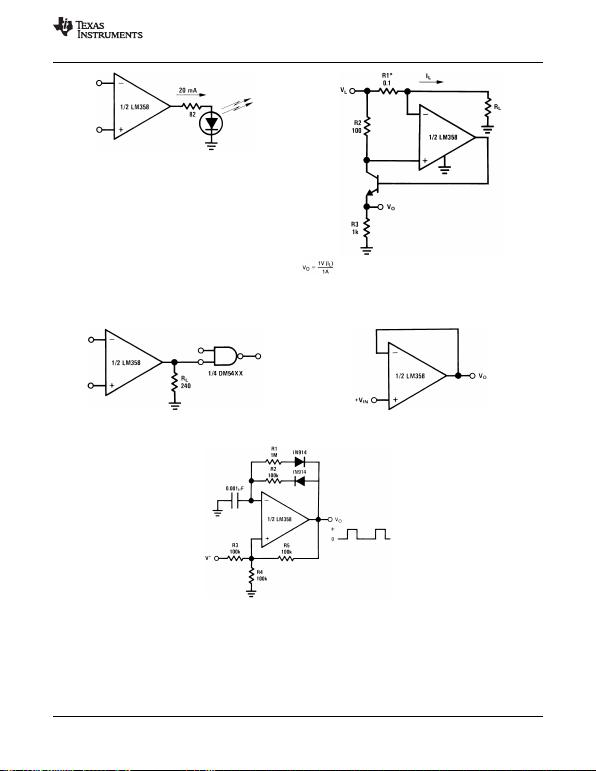LM158QML
www.ti.com
SNOSAP3F JULY 2005REVISED MARCH 2013
*(Increase R1 for ILsmall)
VLV+2V
Figure 22. LED Driver Figure 23. Current Monitor
VO= VIN
Figure 24. Driving TTL Figure 25. Voltage Follower
Figure 26. Pulse Generator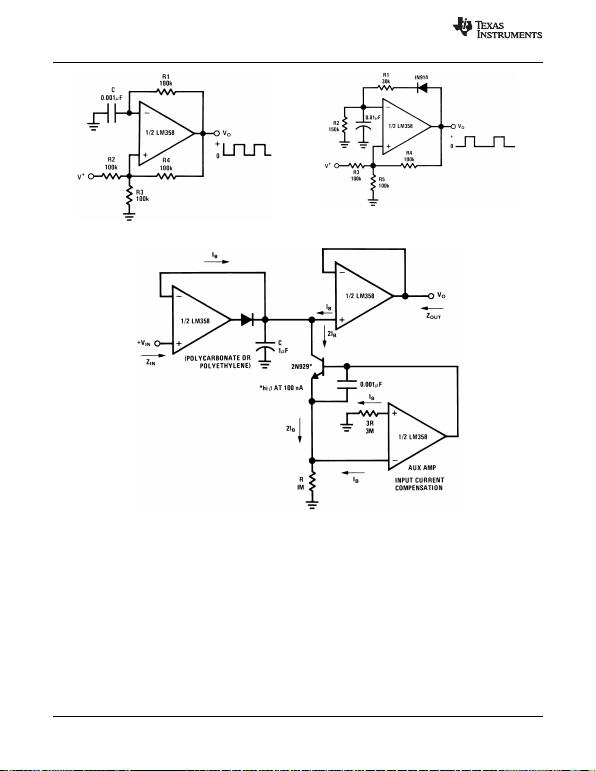LM158QML
SNOSAP3F JULY 2005REVISED MARCH 2013
www.ti.com
Figure 27. Squarewave Oscillator Figure 28. Pulse Generator
HIGH ZIN
LOW ZOUT Figure 29. Low Drift Peak Detector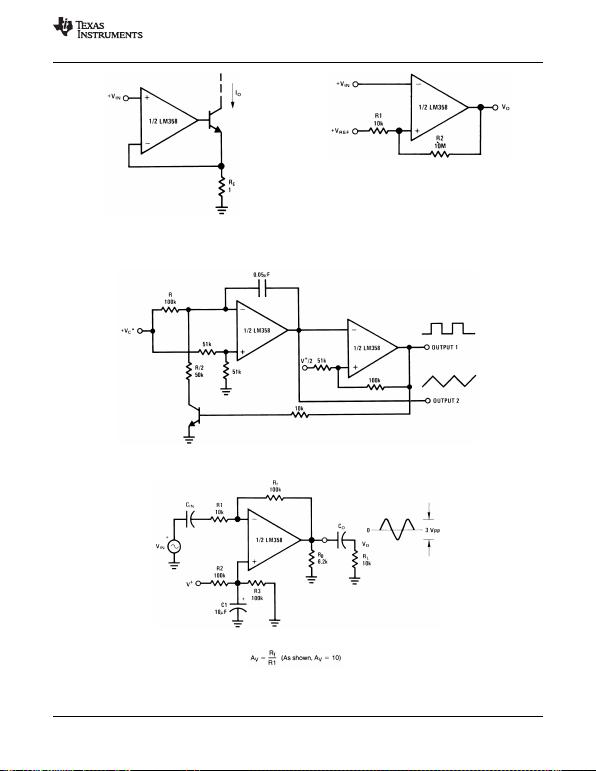LM158QML
www.ti.com
SNOSAP3F JULY 2005REVISED MARCH 2013
IO= 1 amp/volt VIN
(Increase REfor IOsmall)
Figure 30. High Compliance Current Sink Figure 31. Comparator with Hysteresis
*WIDE CONTROL VOLTAGE RANGE: 0 VDC VC2 (V+1.5V DC)
Figure 32. Voltage Controlled Oscillator (VCO)
Figure 33. AC Coupled Inverting Amplifier